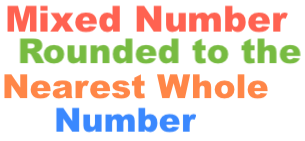4 1/6 Rounded to the Nearest Whole NumberHere we will show you step-by-step how to get the solution to: "What is 4 1/6 rounded to the nearest whole number?"

4 1/6 is a mixed number with a whole number and a fraction. We want to round to the nearest whole number without the fraction.

First, we convert the mixed fraction to an improper fraction or fraction like this:

((4 x 6)+1)/6 = 25/6

Then, we divide the numerator by the denominator from the previous step:

25/6 = 4.16

Now we have one decimal number. Round using the following rules:

If the number to the right of the decimal point is .5 or higher, then add 1 to the left of the decimal point.

If the number to the right of the decimal point is less than .5, then leave the number to the left of the decimal point as is.

.16 is less than .5. Thus, we leave the number to the left of the decimal point as is. 4 1/6 rounded to the nearest whole number is therefore:

4

Mixed Number Rounded to the Nearest Whole Number
"What is 4 1/6 rounded to the nearest whole number?" is not the only question we can answer. Round another mixed number here.

What is 4 1/7 rounded to the nearest whole number?
Here is the next mixed number on our list that we have rounded to the nearest whole number.